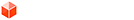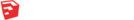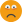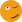## [Code] How do you compute weighted vertex normals?

### [Code] How do you compute weighted vertex normals?

Reference:
http://www.bytehazard.com/code/vertnorm.html
http://meshlabstuff.blogspot.com/2009/0 ... rmals.html

How can you compute weighted vertex normals for vertices in SketchUp?

Vertex Normal.png

Compute vertex normals so these two boxes produces similar normals.
Adding up all connected face normals results in a normal that lean towards the triangulated side - which not what I want.

Current Solution
The current solution that appear to yield the best results:
Code: Select all
`  def self.vertex_normal( vertex )    faces = vertex.faces    edges = vertex.edges    point = vertex.position    normal = Geom::Vector3d.new( 0, 0, 0 )    until faces.empty?      face = faces.shift      e1, e2 = face.edges & edges      # Ensure the vectors are in the order of the loop.      test_vertex = e1.reversed_in?( face ) ? e1.start : e1.end      if test_vertex == vertex        e1, e2 = [ e2, e1 ]      end      # Now the full angle can be calculated.      pt1 = e1.other_vertex( vertex ).position      pt2 = e2.other_vertex( vertex ).position      v1 = point.vector_to( pt1 )      v2 = point.vector_to( pt2 )      angle = self.full_angle_between( v1, v2, face.normal )      face_normal = face.normal      face_normal.length = angle      normal += face_normal    end    normal.normalize!  end    def self.full_angle_between( vector1, vector2, normal )    cross = vector1 * vector2    direction = cross % normal    angle = vector1.angle_between( vector2 )    angle = 360.degrees - angle if direction > 0.0    angle  end`
0
Last edited by thomthom on Thu Aug 25, 2011 12:04 pm, edited 7 times in total.
Thomas Thomassen — SketchUp Monkey & Coding addictthomthom
PluginStore Author### Re: How do you computer weighted vertex normals?

Perhaps something like
faces = vertex.faces
return nil if not faces
norm = faces.normal
faces.shift
faces.each{|face| norm = norm + face.normal }
return norm

???
EDIT: Fixed typo * >>> + TIG.
0
TIGTIG
Global Moderator

### Re: How do you computer weighted vertex normals?

That's the naive version I used. But it doesn't always produce the normal one want. The linked articles describe the issues that method has, and further explains one has to weigh the contribution of the faces.
0
Thomas Thomassen — SketchUp Monkey & Coding addictthomthom
PluginStore Author### Re: How do you computer weighted vertex normals?

Well then try perhaps 'weight' by each face's surface area...
faces = vertex.faces
return nil if not faces
norm = faces.normal
norm.length = faces.area
faces.shift
faces.each{|face|
fnor = face.normal
fnor.length = face.area
norm = norm + fnor
}
norm.normalize!
return norm

OR get relative angles between the normals and make adjustments ???
EDIT: typo fixed * >>> + TIG.
0
TIGTIG
Global Moderator

### Re: How do you computer weighted vertex normals?

That snippet gave me zero length vectors for a vertex with three connected faces at an corner.
0
Thomas Thomassen — SketchUp Monkey & Coding addictthomthom
PluginStore Author### Re: How do you computer weighted vertex normals?

Wouldn't it have something to do with the angle between the 2 edges of the face at that vertex * that face's normal. Something so that a small sliver of a face - only 5 degrees for example, would get its normal * 5. Then a face with 90 degrees would get its normal weight * 90. Then combine all those normals, and the vector will be greater than 1, so normalize it to scale it back down.

Would that give an appropriate weight to each face in the overall vertex normal?
0
Lately you've been tan, suspicious for the winter.
All my Plugins I've writtenChris Fullmer
SketchUp Team### Re: How do you computer weighted vertex normals?

Chris Fullmer wrote:Wouldn't it have something to do with the angle between the 2 edges of the face at that vertex * that face's normal. Something so that a small sliver of a face - only 5 degrees for example, would get its normal * 5. Then a face with 90 degrees would get its normal weight * 90. Then combine all those normals, and the vector will be greater than 1, so normalize it to scale it back down.

Would that give an appropriate weight to each face in the overall vertex normal?

What you describe is one of the methods in the links in my OP. The problem is that I don't fully understand how to implement the weighting...0
Thomas Thomassen — SketchUp Monkey & Coding addictthomthom
PluginStore Author### Re: How do you computer weighted vertex normals?

This appear to work:
Code: Select all
`def self.vertex_normal( vertex )    faces = vertex.faces    edges = vertex.edges    point = vertex.position    return nil if faces.empty?    normal = Geom::Vector3d.new( 0, 0, 0 )    until faces.empty?      face = faces.shift      e1, e2 = face.edges & edges      pt1 = e1.other_vertex( vertex ).position      pt2 = e2.other_vertex( vertex ).position      v1 = point.vector_to( pt1 )      v2 = point.vector_to( pt2 )      angle = v1.angle_between( v2 )      face_normal = face.normal      face_normal.length = angle      normal += face_normal    end    normal.normalize!  end`
0
Thomas Thomassen — SketchUp Monkey & Coding addictthomthom
PluginStore Author### Re: How do you computer weighted vertex normals?

Though, the linked article seem to also take into account the face area... wonder what importance that has...
0
Thomas Thomassen — SketchUp Monkey & Coding addictthomthom
PluginStore Author### Re: How do you computer weighted vertex normals?

hmm... it must be flawed. if a face is connected to the vertex then vector1.angle_between( vector2 ) won't give the correct angle, as it only return angles between 0 - 180 degrees. Either I need to deal with each triangle in each face, or I calculate the full angle.
0
Thomas Thomassen — SketchUp Monkey & Coding addictthomthom
PluginStore Author### Re: How do you computer weighted vertex normals?

Stupid typo is my code should be norm + fnor not '*'... I've corrected the original - it should now work as expected - weighting vertex-normals dependent on the face's areas!
0
TIGTIG
Global Moderator

### Re: How do you computer weighted vertex normals?

This seem to account for wide angle corners:

Code: Select all
`  def self.vertex_normal( vertex )    faces = vertex.faces    edges = vertex.edges    point = vertex.position    normal = Geom::Vector3d.new( 0, 0, 0 )    until faces.empty?      face = faces.shift      e1, e2 = face.edges & edges      pt1 = e1.other_vertex( vertex ).position      pt2 = e2.other_vertex( vertex ).position      v1 = point.vector_to( pt1 )      v2 = point.vector_to( pt2 )      angle = self.full_angle_between( v1, v2, face.normal )      face_normal = face.normal      face_normal.length = angle      normal += face_normal    end    normal.normalize!  end    def self.full_angle_between( vector1, vector2, normal )    cross = vector1 * vector2    direction = cross % normal    angle = vector1.angle_between( vector2 )    angle = 360.degrees - angle if direction > 0.0    angle  end`
0
Thomas Thomassen — SketchUp Monkey & Coding addictthomthom
PluginStore Author### Re: How do you computer weighted vertex normals?

TIG wrote:Stupid typo is my code should be norm + fnorm not '*'... I've corrected the original - it should now work as expected !!!!

I'll revisit your code again. http://www.bytehazard.com/code/vertnorm.html mentions that using the area still makes some faces weigh too much, but that it's not that notable. But since it looks to require less computing that checking the angles then it might be preferable.
0
Thomas Thomassen — SketchUp Monkey & Coding addictthomthom
PluginStore Author### Re: How do you computer weighted vertex normals?

hm... I added face area to the equations, but that yielded deviating normals depending how I divided the large face.
removing the face area appear to yield the exact same result.

VertexNormals.png

Weighted by angle:
1. Vector3d(0.419345, 0.0772543, 0.904534)
2. Vector3d(0.419345, 0.0772543, 0.904534)
3. Vector3d(0.419345, 0.0772543, 0.904534)

Weighted by area * angle:
1. Vector3d(0.324298, 0.116468, 0.938758)
2. Vector3d(0.706956, 0.253896, 0.660114)
3. Vector3d(0.770689, 0.276785, 0.573959)
0
Thomas Thomassen — SketchUp Monkey & Coding addictthomthom
PluginStore Author### Re: [Code] How do you computer weighted vertex normals?

I wonder if the linked article uses the face area because they computer the normals for lighting...
0
Thomas Thomassen — SketchUp Monkey & Coding addictthomthom
PluginStore Author### Re: [Code] How do you computer weighted vertex normals?

Several coplanar faces at a vertex are the same as one face of the same area as the bits? So the both area-weight-adjusted vertex-normals will be the same.
I think it is more for lighting...
0
TIGTIG
Global Moderator

### Re: [Code] How do you computer weighted vertex normals?

TIG wrote:Several coplanar faces at a vertex are the same as one face of the same area as the bits? So the both area-weight-adjusted vertex-normals will be the same.

But if a vertex is connected to three sides, one large and two small, then using the area would make the normal lean toward the large area, would it not?
0
Thomas Thomassen — SketchUp Monkey & Coding addictthomthom
PluginStore Author### Re: [Code] How do you computer weighted vertex normals?

Not if the two small areas were equivalent to the large one... then it'd be 'balanced'...
The large area pulls it over then the 1st small are pulls it back and then the 2nd small area pulls it back again.
IF there's a large and a small area the large one 'wins'.
0
TIGTIG
Global Moderator

### Re: [Code] How do you computer weighted vertex normals?

By computer you mean compute?
0
SuSolid.com - solid check - solid repair- solid intersection check - weight pluginvoljanko
PluginStore Author### Re: [Code] How do you computer weighted vertex normals?

voljanko wrote:By computer you mean compute?
A simple typo by tt in the original title - but we understood what he meant...0
TIGTIG
Global Moderator

### Re: [Code] How do you computer weighted vertex normals?

I'm trying to follow your conversation,but not sure to understand what are you trying to do.
Do you want to align faces that are nearly aligned?
0
SuSolid.com - solid check - solid repair- solid intersection check - weight pluginvoljanko
PluginStore Author### Re: [Code] How do you computer weighted vertex normals?

TIG wrote:Not if the two small areas were equivalent to the large one... then it'd be 'balanced'...
The large area pulls it over then the 1st small are pulls it back and then the 2nd small area pulls it back again.
IF there's a large and a small area the large one 'wins'.

Yes, but when they are not, which would be in any non-regular mesh. So I still wonder why one would use area to weigh the normals.
0
Thomas Thomassen — SketchUp Monkey & Coding addictthomthom
PluginStore Author### Re: [Code] How do you computer weighted vertex normals?

TIG wrote:
voljanko wrote:By computer you mean compute?
A simple typo by tt in the original title - but we understood what he meant...Fixed!0
Thomas Thomassen — SketchUp Monkey & Coding addictthomthom
PluginStore Author### Re: [Code] How do you computer weighted vertex normals?

voljanko wrote:I'm trying to follow your conversation,but not sure to understand what are you trying to do.
Do you want to align faces that are nearly aligned?

No, I just want to compute the vertex normals.
In this particular case I need it to be able to calculate some of the topographical characteristic of the mesh.
0
Thomas Thomassen — SketchUp Monkey & Coding addictthomthom
PluginStore Author### Re: [Code] How do you compute weighted vertex normals?

My full_angle_between code seem to not work in all scenarios... back to the drawing table...
0
Thomas Thomassen — SketchUp Monkey & Coding addictthomthom
PluginStore Author### Re: [Code] How do you compute weighted vertex normals?

Now I seemed to have corrected it. I had to ensure the vectors I used came in the same direction as the edge loop.
0
Thomas Thomassen — SketchUp Monkey & Coding addictthomthom
PluginStore Author